• Register
Devblog 9 - Generating a terrain based on bricks - Chapter 1

Let's see, something that I would like this game to have is terrain to explore like Minecraft, with its caves, mountains, seas, etc.As I had already told at the beginning, to do something like this, first we have to generate a three-dimensional matrix in which we decide what type each block is (earth, water, air, etc.)

Posted by on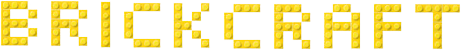Let's see, something that I would like this game to have is terrain to explore like Minecraft, with its caves, mountains, seas, etc.
As I had already told at the beginning, to do something like this, first we have to generate a three-dimensional matrix in which we decide what type each block is (earth, water, air, etc.)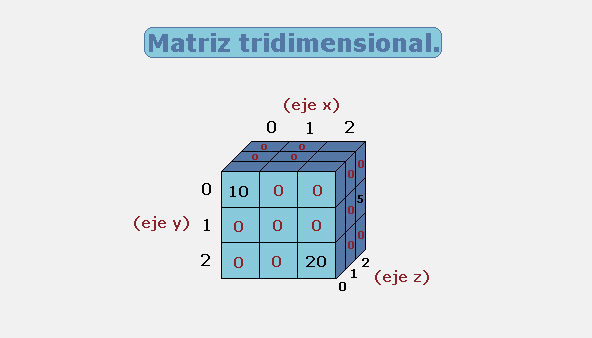It is something that I did not want to do at first because in the world of bricks, there are more shapes than squares. But screw it up, I'll only use 1 square brick model to generate the whole environment.
Now that we have given up on our (block) diversity principles we can move on.

Once we have generated the matrix, we have the most complicated part, rendering it.
We cannot render it whole, there would be no GPU capable of loading so many bricks (and it would make no sense), we must opt ​​for a chunk system.
This is nothing more than dividing the matrix, into smaller parts. So we can load or unload them as the player moves around the map.
This would be a chunk, an area delimited by "x", "z" and not by "y":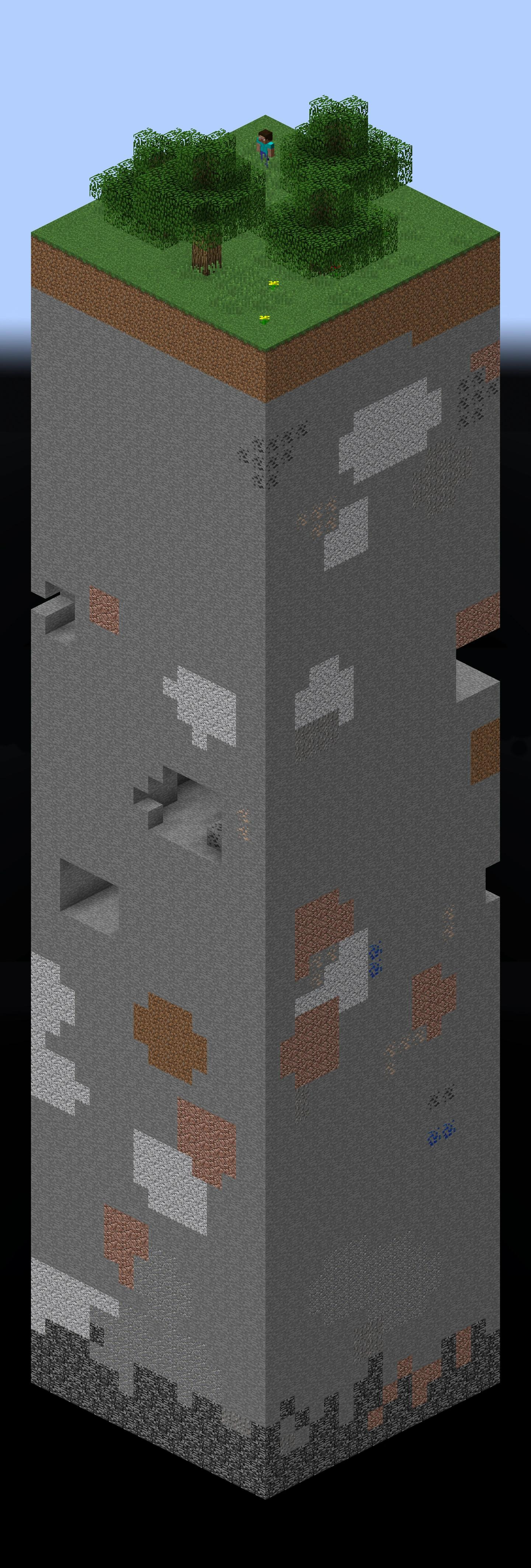With this subdivision we must now worry about understanding which blocks are "exposed". See where the player is in that image.
What's the point of rendering the blocks after the ground if you can't see them?
We must determine which blocks are exposed and render only these: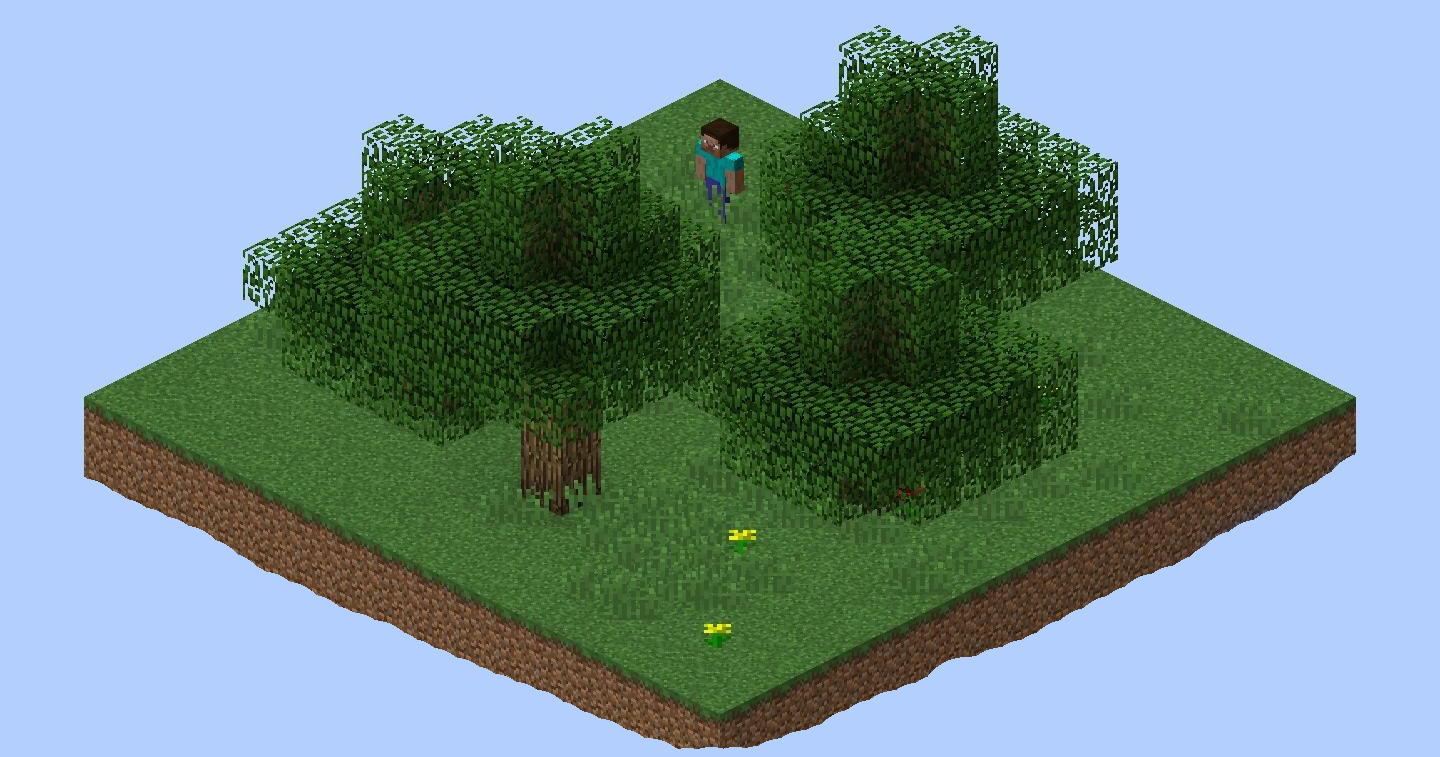And theeen, we have to put the focus on the model itself. A square has multiple faces, the player will rarely see all of them (water blocks). For example, from this angle, only 3 are visible: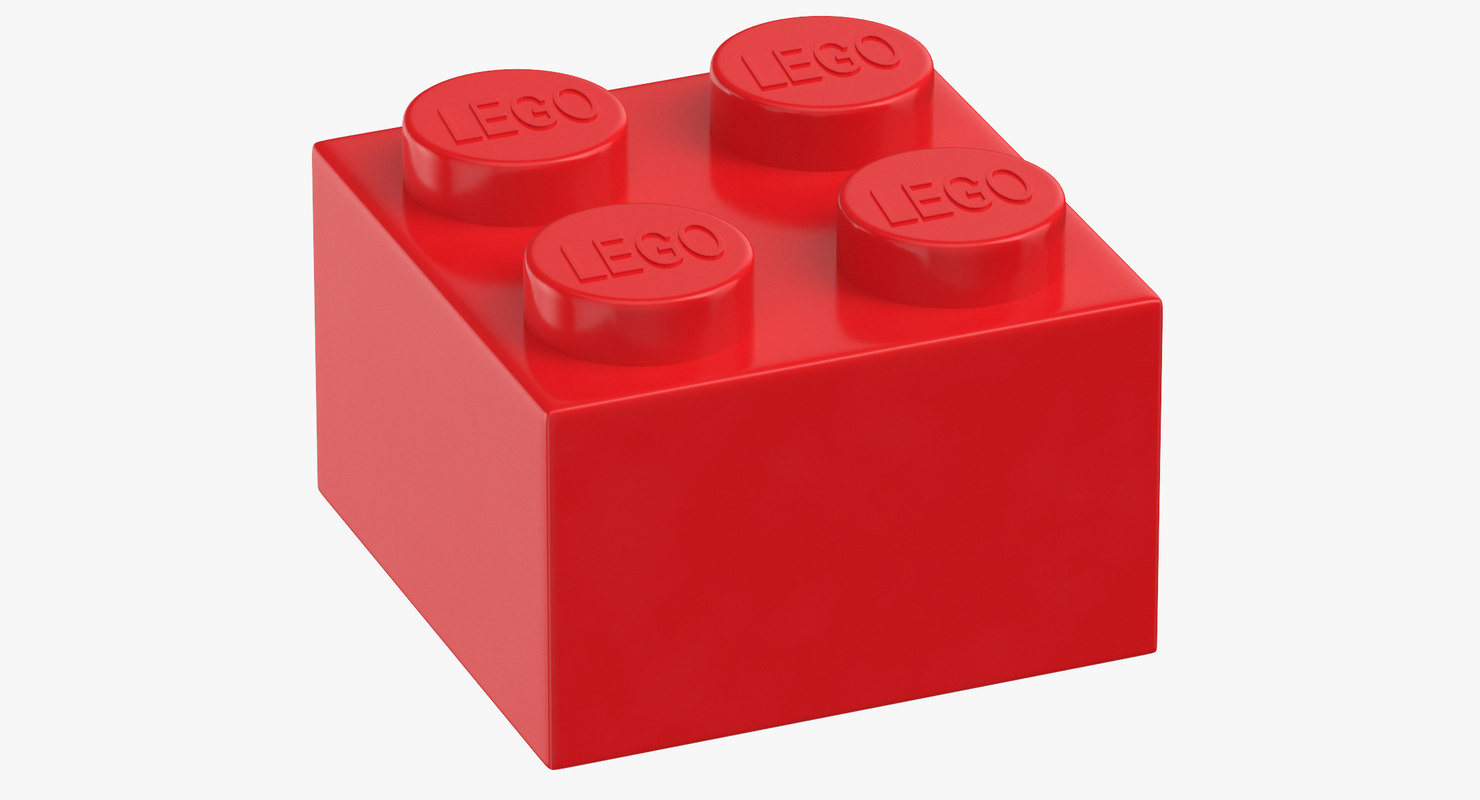And not even that, the blocks are going to be surrounded by other blocks, so it is silly that we render the entire model.

To summarize then:
We need to generate a matrix, divide it by chunks, in each chunk determine the exposed blocks and in each exposed block, determine the visible faces.
Once we know this, we must grab said face from the model and join it in a new model in which we will only put all the exposed faces of the chunk.
To cut the face of a model, it is necessary to understand how a mesh / model works.

### Understanding meshes / meshes

A mesh is a set of points (vertices) that, connected to each other, form triangles. To know where each triangle is looking / painted we use vectors (normals) and finally we also map how the material (texture) is applied to the mesh.
Okay, my explanation may not be the best. Let's try a more visual example.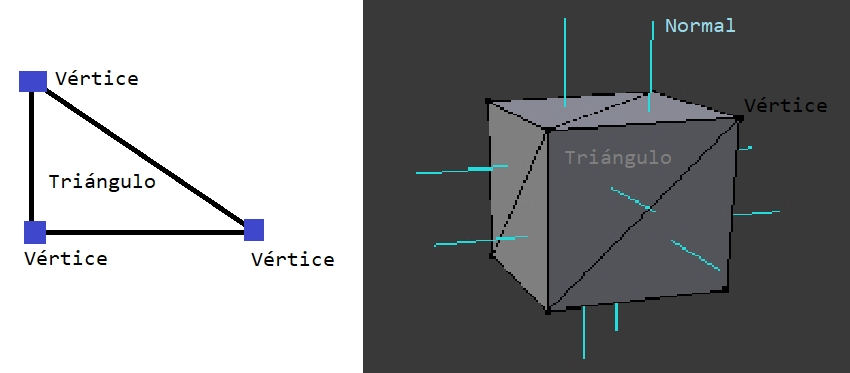The face of a cube is a square, which is made up of 2 triangles. Each one created by 3 vertices out of a total of 4 that has the entire face.
If we wanted to make the face of a square in Unity (ignoring where the triangles are facing and what color they are) we would do this: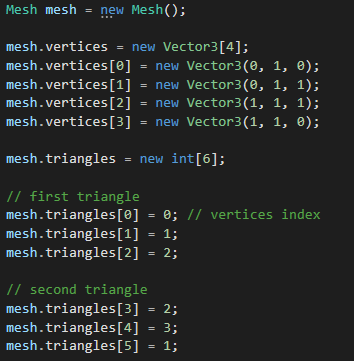How do we determine which triangles make up a face of the mesh?
Good question, I have no idea. But I can think of a trick to get out of the way.
Look at the shape of a basic brick:In this case, to know the triangles of each face, what we can do is add a plane that acts as a "delimiter", any vertex that is after said limit will be part of the face in question.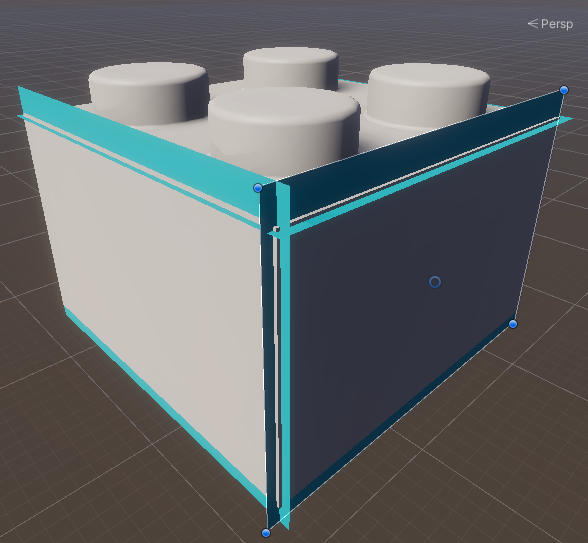If you notice, each blue plane is just before the end of a face.
Well, now we have to code something that will iterate all the triangles of the mesh with each delimiter to know which face they belong to.
In Unity we have the mesh data in the following format:

``````int [] triangles;
Vector3 [] vertices;``````

The triangles are pointed by a list of integers, which only refer to the list of vertices:

``````int[] # which would look like: [0,1,2,2,3,0]
Vector3[]
[
0 => Vector3(0, 0, 0),
1 => Vector3(0, 0, 1),
2 => Vector3(1, 0, 1),
3 => Vector3(1, 0, 0)
]```Maybe it's easier to visualize it:```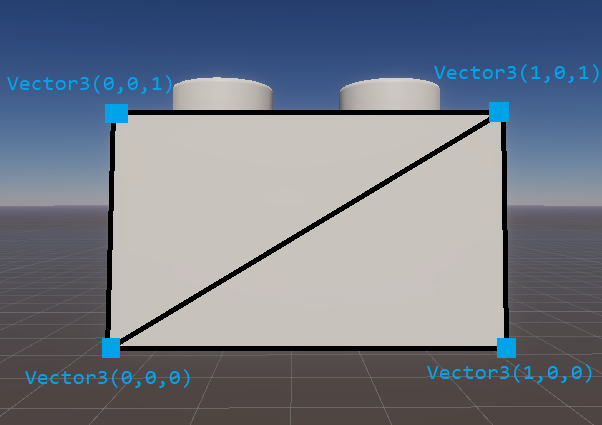As we already know, each triangle is made up of 3 vertices, so to obtain a triangle from the list of triangles, each time we will take 3 values:
- The first triangle will be 0,1,2
- The second 2,3,0
These are just the indices to take from the list of vertices, so now we know that the first triangle, the one on the left, is made up of the vertices:
0 => Vector3 (0, 0, 0)
1 => Vector3 (0, 0, 1)
2 => Vector3 (1, 0, 1)
And the second:
2 => Vector3 (1, 0, 1)
3 => Vector3 (1, 0, 0)
0 => Vector3 (0, 0, 0)
Which you should be able to easily corroborate with the image I have posted.
We do a quick test to get the triangles for each face: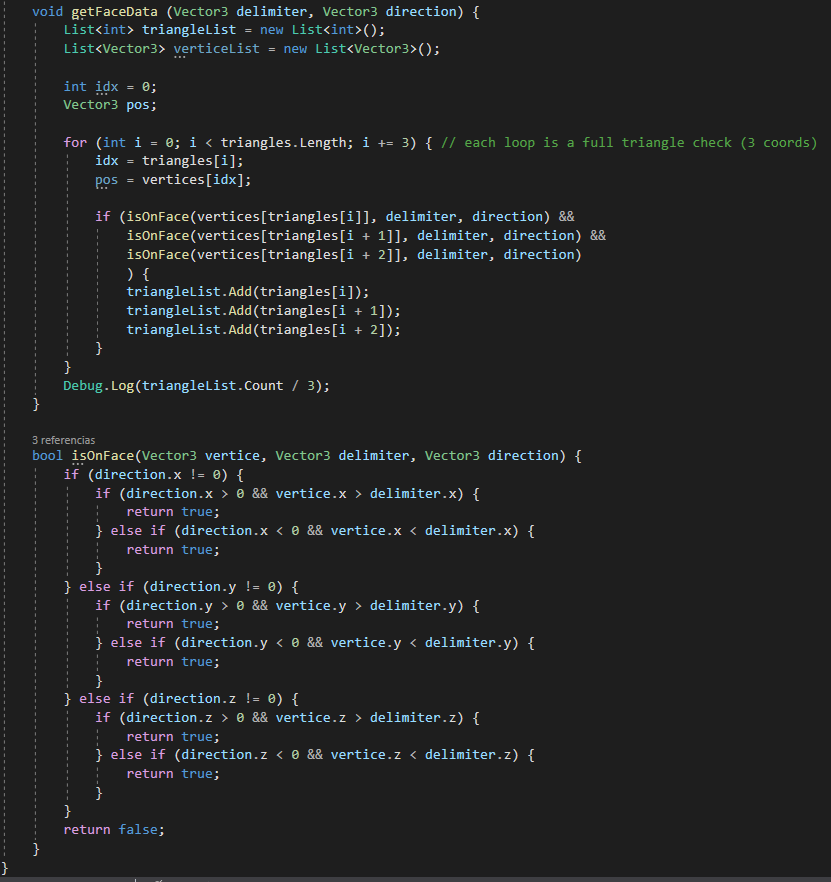And ........... W-T-F ..
These are the results:
Top face: 784
Bottom face: 80
Left face: 8
Right face: 8
Front face: 4
Back face: 4

It is normal for the upper face to have a lot of triangles because it has the studs (but it still has too many). The one below .. well look because it has the round ones from the clutch.

But ... What the hell is wrong with the side faces?????????
We are talking about a cube, how can they have different number of triangles per side? and how could it be bigger than 2!!!??

It is clear that I have done something wrong, so I create a quick script to paint the vertices directly on the model and ................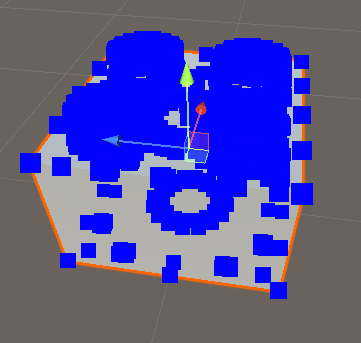I'ts not easy to understand because the upper face, with the studs, is full of vertices. And the bottom circle of the piece is also easily seen.

If we "tear off" the sides of the faces we see that no, my system to separate triangles works correctly: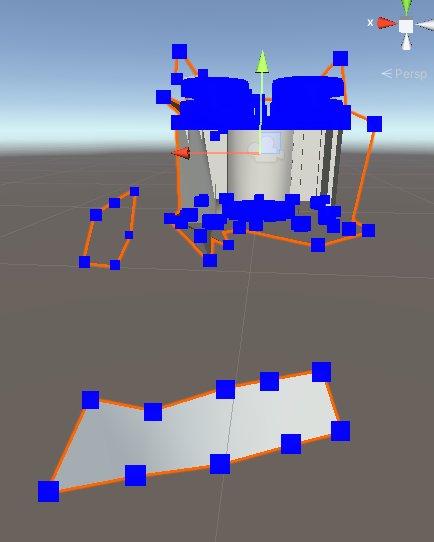One of the faces has 8 triangles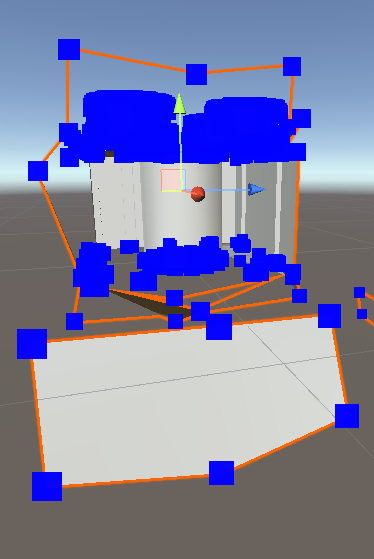Another we see that 4.
I remind you that we are counting triangles: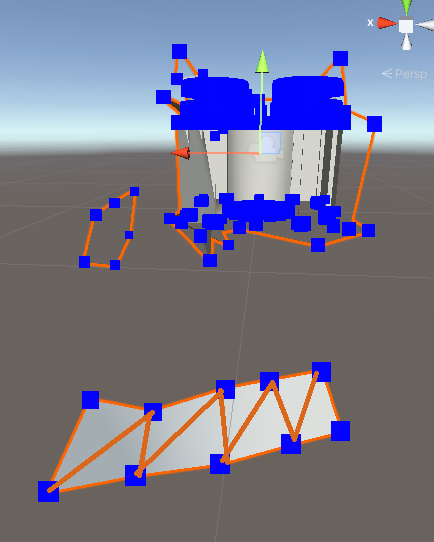So the problem is the model itself, it has more triangles than it really needs.

The only way to fix it is by optimizing the model, opening it in some 3d editor like Blender and removing triangles. The thing is, I have no idea about modeling. So here I am, trapped.
Let's see if I can find someone to ask to touch up the model.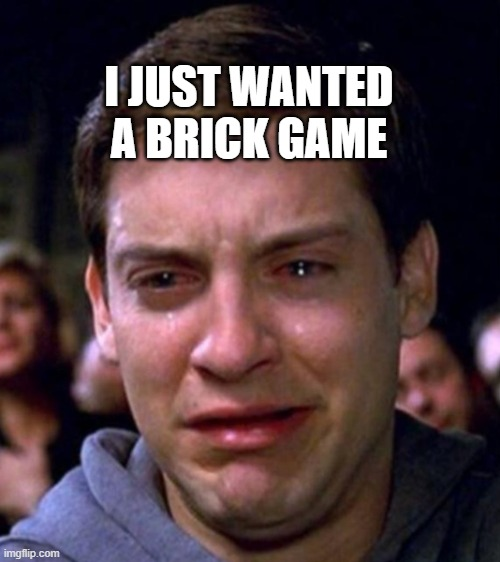Github.com